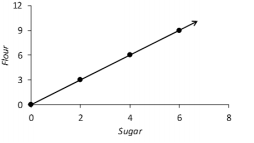##### Eureka Math Module 1

0
Set Details Share
created 8 days ago by MrsShoopman
1 view
updated 8 days ago by MrsShoopman
Grade levels:
7th grade
Subjects:
math 7
show moreless
Page to share:
Embed this setcancel
COPY
code changes based on your size selection
Size:
X
1

Constant of Proportionality

(If a proportional relationship is described by the set of ordered pairs that satisfies the equation y=kx, where k is positive constant, then k is called the constant of proportionality. For example, if the ratio of y to x is 2 to 3, then the constant of proportionality is 2/3 and y =2/3x)

2

Miles per Hour

(One mile per hour is a proportional relationship between d miles and t hours given by the equation d=1t (both d and t are positive real numbers). Similarly, for any positive real number v, v miles per hour is a proportional relationship between d miles and t hours given by d=vt. The unit for the rate, mile per hour (or mile/hour) if often abbreviated as mph)

3

Coodrinate Plane4

Ratio Table